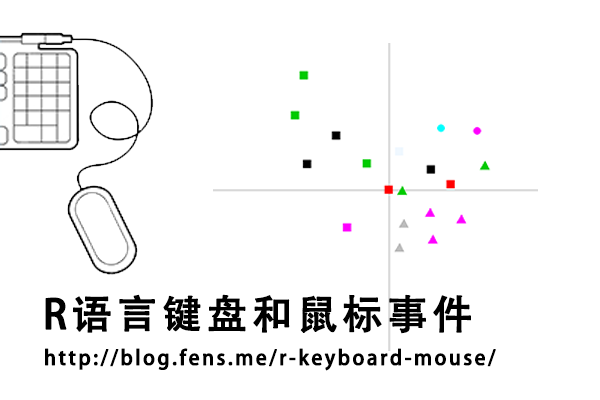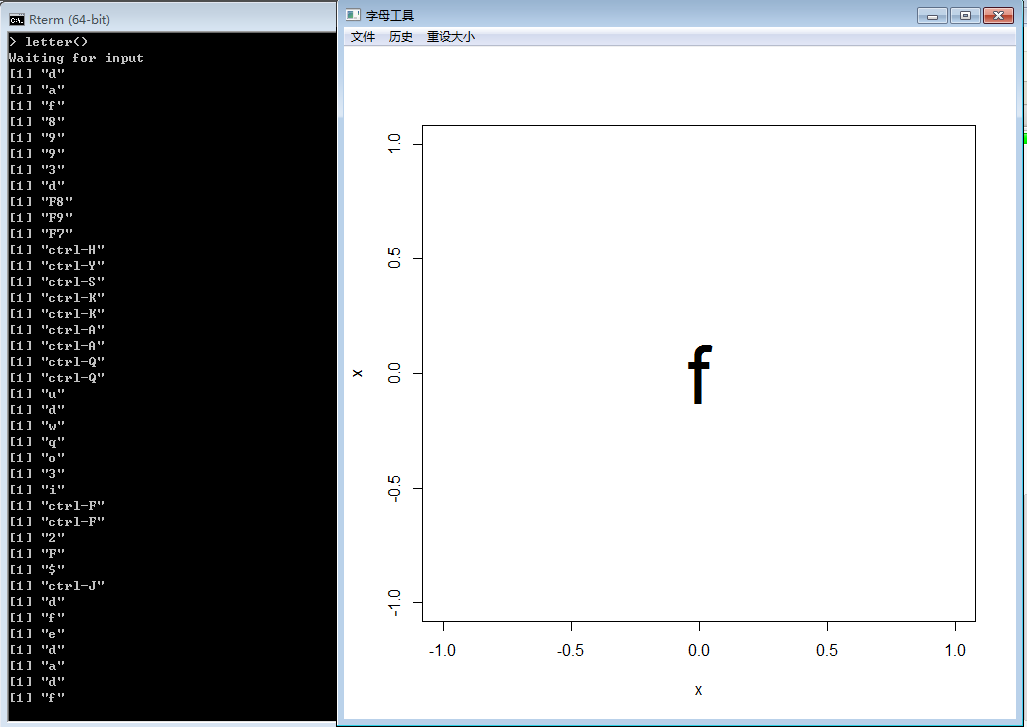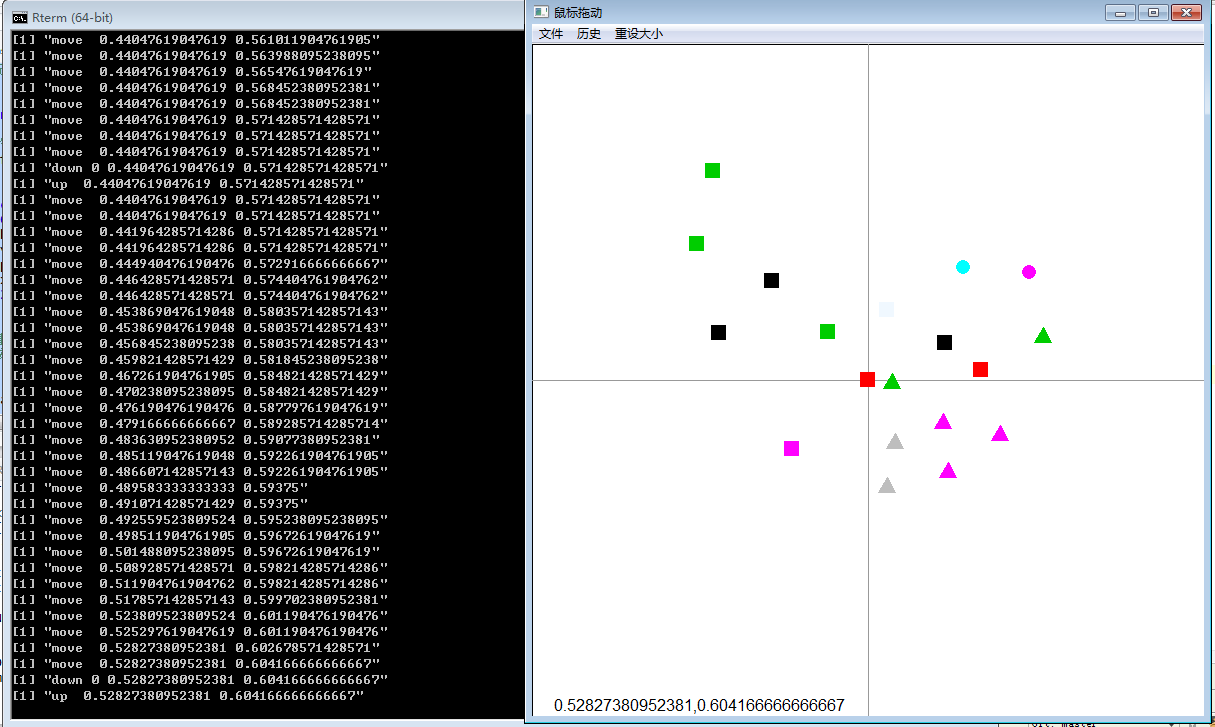• Posts tagged "event"

# Blog Archives

## R语言键盘和鼠标事件

R的极客理想系列文章，涵盖了R的思想，使用，工具，创新等的一系列要点，以我个人的学习和体验去诠释R的强大。

R语言作为统计学一门语言，一直在小众领域闪耀着光芒。直到大数据的爆发，R语言变成了一门炙手可热的数据分析的利器。随着越来越多的工程背景的人的加入，R语言的社区在迅速扩大成长。现在已不仅仅是统计领域，教育，银行，电商，互联网….都在使用R语言。

• 张丹(Conan), 程序员Java,R,PHP,Javascript
• weibo：@Conan_Z
• blog: http://blog.fens.me
• email: bsspirit@gmail.com1. R语言图形事件
2. 图形事件API
3. 键盘事件
4. 鼠标事件

## 1. R语言图形事件

R语言中，应用最广的一类功能是画图，我们会经常使用R语言作图的API，像plot(),barplot(),points(),lines()等。每次调用plot()函数，R运行时环境都会启动一个新的图形设备(Graphics Device)。这个新打开的图形设备，除了会显示我们的定义的图形，还内置了一套事件管理器，可以监听键盘和鼠标事件，实现与图形设备的通信。

## 2. 图形事件API

• setGraphicsEventHandlers, 注册图形事件，包括键盘事件和鼠标事件(按下，释放，移动)。
• getGraphicsEvent, 启动图形事件监听器，开始监听。
• setGraphicsEventEnv, 设置图形设备和环境空间，默认为当前图形设备和当前环境空间。
• getGraphicsEventEnv, 获取图形设备，默认为当前图形设备。

``````
> setGraphicsEventHandlers
function (which = dev.cur(), ...)
setGraphicsEventEnv(which, as.environment(list(...)))
<bytecode: 0x000000000b321d20>
<environment: namespace:grDevices>
``````

``````
> getGraphicsEvent
function (prompt = "Waiting for input", onMouseDown = NULL, onMouseMove = NULL,
onMouseUp = NULL, onKeybd = NULL, consolePrompt = prompt)
{
if (!interactive())
return(NULL)
if (!missing(prompt) || !missing(onMouseDown) || !missing(onMouseMove) ||
!missing(onMouseUp) || !missing(onKeybd)) {
setGraphicsEventHandlers(prompt = prompt, onMouseDown = onMouseDown,
onMouseMove = onMouseMove, onMouseUp = onMouseUp,
onKeybd = onKeybd)
}
.External2(C_getGraphicsEvent, consolePrompt)
}
<bytecode: 0x0000000005c4bd40>
<environment: namespace:grDevices>
``````

• prompt, 文字提示
• consolePrompt, 在终端中的文字提示
• onMouseDown, 鼠标事件，按下鼠标按键
• onMouseUp, 鼠标事件，释放鼠标按键
• onMouseMove, 鼠标事件，鼠标移动
• onKeybd, 键盘事件，按下键盘

## 3. 键盘事件

• Win7 64bit
• R: 3.0.1 x86_64-w64-mingw32/x64 b4bit

• 1. 利用R语言画图，根据键盘输入显示对应的文字。
• 2. 每按一下键盘，输出一个字母在屏幕上面。
• 3. 按ctrl+c时，停止键盘事件。``````
# 字母工具
letter<-function(){

# 画图函数
draw<-function(label='',x=0,y=0){
plot(x,y,type='n')
text(x,y,label=label,cex=5)
}

# 键盘事件
keydown<-function(K){
if (K == "ctrl-C") return(invisible(1))
print(K)
draw(K)
}

# 画图
draw()

# 注册键盘事件，启动监听
getGraphicsEvent(prompt="Letter Tool",onKeybd = keydown)
}

#启动程序
letter()
``````

## 4. 鼠标事件

R语言中，图形设备的鼠标事件，包括3种类型： 鼠标移动， 鼠标按下(左键，右键，滚轮)，鼠标释放。

• 1. 利用R语言画图，根据鼠标事件指定图形的位置和形状。
• 2. 每移动一下鼠标，左下角输出鼠标所在的坐标，同时一个默认的图形随鼠标移动。
• 3. 当鼠标按下时，会在当前坐标出现一个图形，左键对应正方形，滚轮对应用圆形，右键对应三角形。
• 4. 按q时，停止监听事件。``````
mouse <- function() {
# 设置画布无边
par(mai=rep(0,4),oma=rep(0,4))

# 初始化的点
ps<-data.frame(x=c(0.5),y=c(0.5),col=c(2),pch=c(15))

# x,y为鼠标坐标
draw<-function(x=0,y=0){
plot(0,0,xlim=c(0,1),ylim=c(0,1),type='n',xaxs="i", yaxs="i")
abline(h=0.5,col="gray60") # 水平线
abline(v=0.5,col="gray60") # 垂直线
points(ps\$x,ps\$y,pch=ps\$pch,cex=2,col=ps\$col) # 实点标点
points(x,y,pch=15,cex=2,col=colors()[ps\$col]) # 鼠标坐标点
text(0.25,0.015,label=paste(x,y,sep=",")) # 鼠标坐标
}

# 鼠标按键监听
# 当鼠标按键，会增加一个实点
# buttons,0是左键,1是滚轮,2是右键
mouseDown <- function(buttons, x, y) {
print(paste("down",buttons,x,y))

# 形状设置
shape<-15
if(buttons==1) shape<-16
if(buttons==2) shape<-17

# 增加实点
ps<<-rbind(ps,data.frame(x=c(x),y=c(y),pch=c(shape),col=round(runif(1,2,500))))
#print(ps)
draw(x,y)
}

# 鼠标移动监听
mouseMove <- function(buttons, x, y) {
print(paste("move",buttons,x,y))
draw(x,y)
}

# 鼠标按键释放监听
mouseup <- function(buttons, x, y) {
print(paste("up",buttons,x,y))
draw(x,y)
}

# 键盘按键监听
keydown <- function(key) {
if (key == "q") return(invisible(1))
}

# 画初始坐标
draw()

# 注册事件
getGraphicsEvent(prompt="mouse",onMouseDown=mouseDown,onMouseMove=mouseMove,onMouseUp=mouseup,onKeybd=keydown)
}

# 启动程序
mouse()
``````